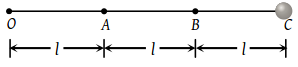A motor cycle driver doubles its velocity when he is having a turn. The force exerted outwardly will be

(1) Double

(2) Half

(3) 4 times

(4) $\frac{1}{4}$ times

Concept Questions :-

Uniform circular motion
High Yielding Test Series + Question Bank - NEET 2020

Difficulty Level:

Two bodies of equal masses revolve in circular orbits of radii R1 and R2 with the same period. Their centripetal forces are in the ratio

(1) ${\left(\frac{{R}_{2}}{{R}_{1}}\right)}^{2}$

(2) $\frac{{R}_{1}}{{R}_{2}}$

(3) ${\left(\frac{{R}_{1}}{{R}_{2}}\right)}^{2}$

(4) $\sqrt{{R}_{1}{R}_{2}}$

Concept Questions :-

Uniform circular motion
High Yielding Test Series + Question Bank - NEET 2020

Difficulty Level:

A mass is supported on a frictionless horizontal surface. It is attached to a string and rotates about a fixed centre at an angular velocity ω0. If the length of the string and angular velocity are doubled, the tension in the string which was initially T0 is now

(1) T0

(2) T0/2

(3) 4 T0

(4) 8 T0

Concept Questions :-

Uniform circular motion
High Yielding Test Series + Question Bank - NEET 2020

Difficulty Level:

Three identical particles are joined together by a thread as shown in figure. All the three particles are moving in horizontal circles centred at O. If the velocity of the outermost particle is v0, then the ratio of tensions in the three sections of the string is(1) 3 : 5 : 7

(2) 3 : 4 : 5

(3) 7 : 11 : 6

(4) 3 : 5 : 6

Concept Questions :-

Uniform circular motion
High Yielding Test Series + Question Bank - NEET 2020

Difficulty Level:

In a circus stuntman rides a motorbike in a circular track of radius R in the vertical plane. The minimum speed at highest point of track will be

(1) $\sqrt{2gR}$

(2) 2gR

(3) $\sqrt{3gR}$

(4) $\sqrt{gR}$

Concept Questions :-

Uniform circular motion
High Yielding Test Series + Question Bank - NEET 2020

Difficulty Level:

A block of mass m at the end of a string is whirled round in a vertical circle of radius R. The critical speed of the block at the top of its swing below which the string would slacken before the block reaches the top is

(1) Rg

(2) (Rg)2

(3) R/g

(4) $\sqrt{Rg}$

Concept Questions :-

Uniform circular motion
High Yielding Test Series + Question Bank - NEET 2020

Difficulty Level:

A bucket tied at the end of a 1.6 m long string is whirled in a vertical circle with constant speed. What should be the minimum speed so that the water from the bucket does not spill, when the bucket is at the highest position (Take g = 10 m/s2

(1) 4 m/sec

(2) 6.25 m/sec

(3) 16 m/sec

(4) None of the above

Concept Questions :-

Uniform circular motion
High Yielding Test Series + Question Bank - NEET 2020

Difficulty Level:

A 1 kg stone at the end of 1 m long string is whirled in a vertical circle at constant speed of 4 m/sec. The tension in the string is 6 N, when the stone is at (g = 10 m/sec2

(1) Top of the circle

(2) Bottom of the circle

(3) Half way down

(4) None of the above

Concept Questions :-

Uniform circular motion
High Yielding Test Series + Question Bank - NEET 2020

Difficulty Level:

The tension in the string revolving in a vertical circle with a mass m at the end which is at the lowest position

(1) $\frac{m{v}^{2}}{r}$

(2) $\frac{m{v}^{2}}{r}-mg$

(3) $\frac{m{v}^{2}}{r}+mg$

(4) mg

Concept Questions :-

Uniform circular motion
High Yielding Test Series + Question Bank - NEET 2020

Difficulty Level:

A coin, placed on a rotating turn-table slips, when it is placed at a distance of 9 cm from the centre. If the angular velocity of the turn-table is trippled, it will just slip, if its distance from the centre is

(1) 27 cm

(2) 9 cm

(3) 3 cm

(4) 1 cm

Concept Questions :-

Uniform circular motion